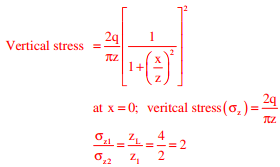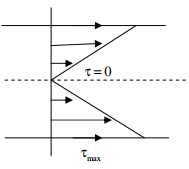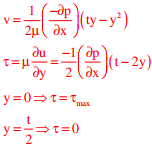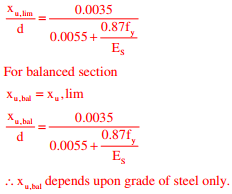# Civil Engineering - Online Test

Q1. Roots of the algebraic equation x3 + x2 + x + 1 = 0 are
Explaination / Solution:Q2. A uniformly distributed line load of 500 kN/m is acting on the ground surface. Based on Boussinesq’s theory, the ratio of vertical stress at a depth 2 m to that at 4 m, right below the line of loading, is
Explaination / Solution:

Due to UDLQ3. Group I lists the type of gain or loss of strength in soils. Group II lists the property or process responsible for the loss or gain of strength in soils.
Group I                                                                        Group II
P. Regain of strength with time                                   1. Boiling
R. Loss of strength due to upward seepage               3. Thixotropy
S. Loss of strength due to remolding                          4. Sensitivity
The correct match between Group I and Group II is

Explaination / Solution:
No Explaination.

Q4. A runway is being constructed in a new airport as per the International Civil Aviation Organization (ICAO) recommendations. The elevation and the airport reference temperature of this airport are 535 m above the mean sea level and 22.65°C, respectively. Consider the effective gradient of runway as 1%. The length of runway required for a design-aircraft under the standard conditions is 2000 m. Within the framework of applying sequential corrections as per the ICAO recommendations, the length of runway corrected for the temperature is
Explaination / Solution:

Q5. For a steady incompressible laminar flow between two infinite parallel stationary plates, the shear stress variation is
Explaination / Solution:

We know that velocity variation,Q6. Which one of the following is NOT present in the acid rain?
Explaination / Solution:
No Explaination.

Q7. The number of spectral bands in the Enhanced Thematic Mapper sensor on the remote sensing satellite Landsat-7 is
Explaination / Solution:
No Explaination.

Q8. The bacteria in milk are destroyed when it _________ heated to 80 degree Celsius.
Explaination / Solution:
No Explaination.

Q9. With K as a constant, the possible solution for the first order differential equationis
Explaination / Solution:Q10. According to IS 456-2000, which one of the following statements about the depth of neutral axis Xu,bal for a balanced reinforced concrete section is correct?
Explaination / Solution:

By limit state method